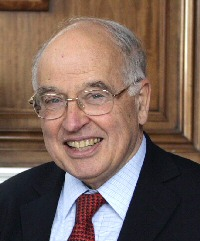A# Atiyah, Michael Francis (1929–)Michael Atiyah is an English mathematician who has contributed to many topics in mathematics, notably dealing with the relationships between geometry and analysis. In topology, he developed K-theory. He proved the index theorem on the number of solutions of elliptic differential equations, linking differential geometry, topology, and analysis – a theorem that has been usefully applied to quantum theory. Atiyah was influential in initiating work on gauge theories and applications to nonlinear differential equations, and in making links between topology and quantum field theory. Theories of superspace and supergravity, and string theory, were all developed using ideas introduced by him.